# Rep-tiles

We want to dissect some shape into congruent shapes, all similar to the initial shape.
Therefore they can tile the plane.

####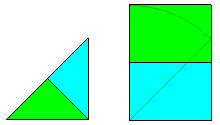Rep-2-tiles

There is just a finite number of regular rep-2-tiles (without turning over) :
• A tan (isosceles right triangle)
• Rectangle with ratio  1:√2 (A4 paper format)

The other ones are fractals ("fractiles") !

• The Levy dragon
• The Heighway dragon
• The twindragons
• The tame twindragons)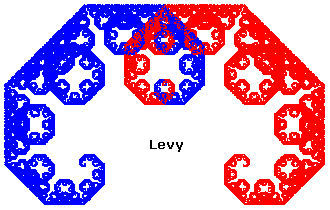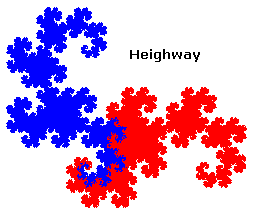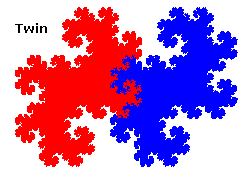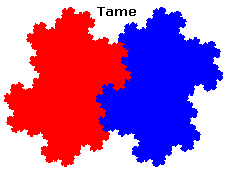And a few others, as weird as the Levy dragon, that is with self intersecting boundary, and quite hard to figure that they don't overlap nor miss some part.
See a more complete zoo.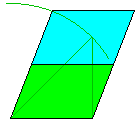With flipping over : the "A4 parallelogram", a parallelogram with side ratio 1:√2

####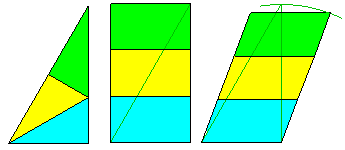Rep-3-tiles

We already saw the 1/2 equilateral triangle (30-60° squares), with flip.
Mention the rectangle with side ratio 1:√3, without flip.
Or with flip, a parallelogram with the same side ratio.
Others are fractals, for instance :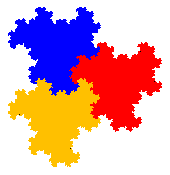####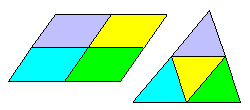Rep-4-tiles

There are much more.
First of all any triangle and any parallelogram is trivially a rep-4-tile.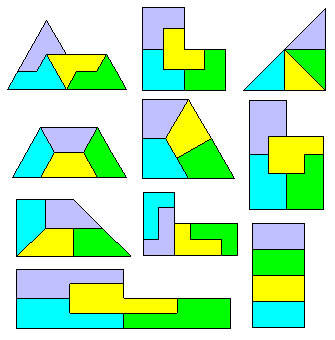Mention the L triomino, the P pentomino, the L tetromino, the isosceles right triangle (with a different dissection than for any triangle) the "sphinx", and some trapezoids.
The L triomino can be "stretched" to build an infinite family of rep-4-tiles (with flip).
The 1×2 rectangle allows a different tiling from the generic parallelogram.

Reaching the -gons, the triangular spiral is a rep-4-tile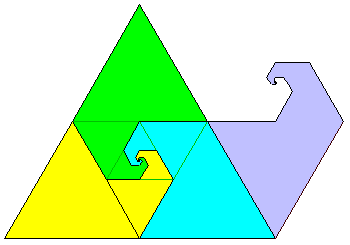Next step is fractal 4-rep-tiles ...

####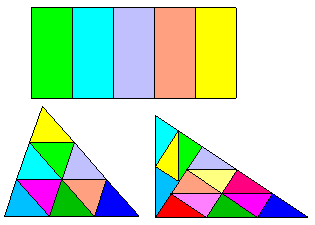And more

Outside specific cases, we have the following rep-tiles families :
• Any rectangle with sides ratio  1:√n is a rep-n-tile
(with flip : a parallelogram with same sides ratio)
• Any triangle is a rep-n²-tile,
• Any right triangle with sides a, b integers is a rep-(a² + b²)-tile.
Pythagorean triangles are then rep-c²-tiles in two different ways.
• At last, any rep-n-tile is of course a rep-n²-tile !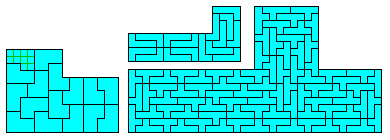There are also many rep-tiles based on polyominoes.
The only pentominoes which are regular rep-tiles are the P (already seen) the L and the Y
The L is "just" a rep-16-tile (and any rep-n²-tile with n>4)
The Y is even worse as it is a rep-81-tile !

Other polyominoes are simpler, for instance this 10-ominoe which is a rep-16-tile.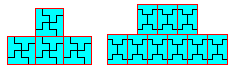Or the L tetromino already seen, rep-4-tile.
Among the tetrominoes, only the T is an "obvious" rep-16-tile.
The same method gives this hexomino as rep-36-tile.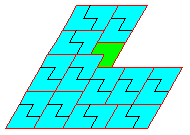Among the "polyamonds" (on a "mesh" of equilateral triangles), we saw the sphinx, which is even a rep-n²-tile for any n. Consider also the "lobster", being a rep-n²-tile for any n = 6k, 6k±1, here rep-5²-tile, as well as the "bat" (120° instead of 60 of the lobster).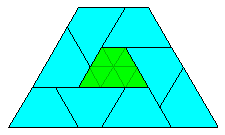A last example is that trapezoid "octiamond" which is a rep-(2n+1)²-tile.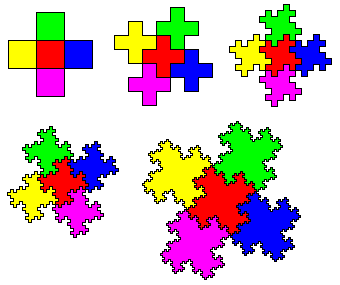We can make fractal rep-n-tiles from a set of polygons.
for instance from 5 squares, we get this fractal rep-5-tile.
We can do the same from 7 hexagons to get a fractal rep-7-tile.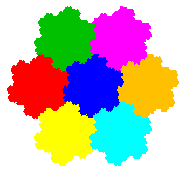Not so far, from the A4 rep-2-tile A4 we can deduce ... the twin dragons !

####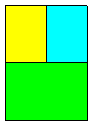Irregular rep-tiles (irrep-tiles)

When the shapes are not congruent but just similar, the rep-tiles are "irregular" (irrep-tiles).
We won't consider further the trivial tiling obtained by replacing one piece of a regular rep-tile by its parts to get a irrep-(2n-1)-tile ! From that point of vue, we get an A4 rectangle is an irrep-3-tile !
We consider then the smallest value of n.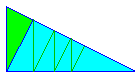Any right triangle is an irrep-2-tile, therefore with the "trivial process" an irrep-n-tile.
(We'll consider with care the resulting "irrep--tile", as any shape is an irrep--tile)An irrep-2-tile with x = φ ≈ 1.272...
Follows a construction from a square ABCD with side 1 :
AE = AB = 1.
The midpoint M of AD gives MF = MC = 5/2, hence AF = φ = x²
The circle with diameter EF intersects AB in H, AH² = AE.AF = φ, hence AH = x.
The parallel FK to EH gives similar triangles AEH and AFK, therefore AK/AF = AH/AE and AK = x³, then completing the outside shape.
The cuts are constructed with UV = AH = x and EW = AF = x²

It seems that these are the only non fractal irrep-2-tiles.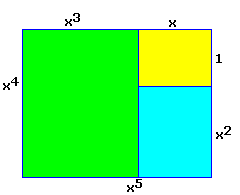The previous irrep-tile gives the rectangular irrep-3-tile :

A not too hard irrep-3-tile :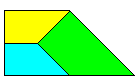But this other irrep-3-tile is much harder :
It is cyclic (opposite right angles in A and C), but the exact construction is not easy.
The following applet allows, for any given value AB/AD, to manually search the suitable point D'.

Sorry, your browser is not Java compliant. Static image.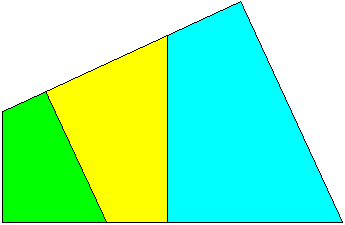However, C is constructed from D' with AC_|_BD' (because of similar triangles and inscribed angles, that is the condition for ABCD to be similar to A'BAD')
The similar quadrilaterals to ABCD are then successively constructed in the angle of lines AD and BC.
(DE1 // AC, E1E2 // BD, E2E3 // AC etc...)
The location of D' is to be adjusted so that the side EiFi of the last quadrilateral is on A'D'.
The "3" et "5" buttons set to predefined irrep-3-tiles and 5-tiles.
Because of the handling of moves in the applet, you may have to repeat the action (move A then D') if A has been changed.
Of course, we can go on and construct any irrep-(2n+1)-tile of this family.
The green points define the base circle and act as a zoom and pan.
A and D' modify the quadrilateral shape.For greater n, there are many more irrep-n-tiles.
A rectangle of side ratio 1: x with x = √(1 + 2) ≈ 1.55377 is an irrep-4-tile.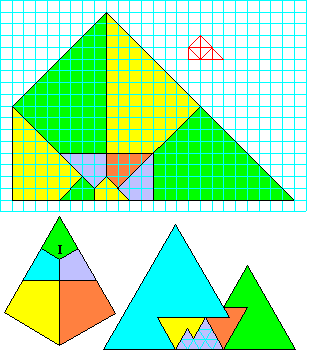More weird irrep-tiles :
The shape made of 7 tans gives an easy constructed irrep-tile on a square grid.

The diamond is easy constructed after noticing that the angles in I are equal, hence 120°.

The mountains are constructed from a triangular grid.

Many irrep-tiles are constructed from various trapezoids, resulting into infinite families.I give here an infinite family of trapezoids, also as an applet to be manually "adjusted".

Sorry, your browser is not Java compliant.
The blue draggable point defines the angle of this trapezoid, the "75°" etc. buttons set it to exact values. The 90° angle obviously gives rectangles !
The numbered buttons choose the rank of the irrep-tile, from 4 à 7. This process can be extended as far as wanted.
B acts as a zoom, C modifies the trapezoid shape. By moving C, we adjust the two cyan and green trapezoids so that they fit in the 'remaining' rectangle.
This adjustment being very inaccurate, the slider to the right gives a fine adjustment of BC/AB.
The filling error of the rectangle by the two trapezoids is displayed in °/oo

This process can be generalized to different fillings of the remaining rectangle by any even number of trapezoids.

There are also fractal irrep-tiles.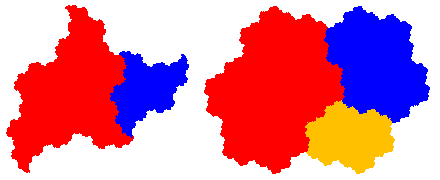A few references:
Paver des pavés by J.P Delahaye (French)
Rep-tiles introduction by S. Dutch
IFS fractal rep-tiles
Polyominoes recreational mathematics archive
mathmagic E. Friedman's Problem of the month oct/2002